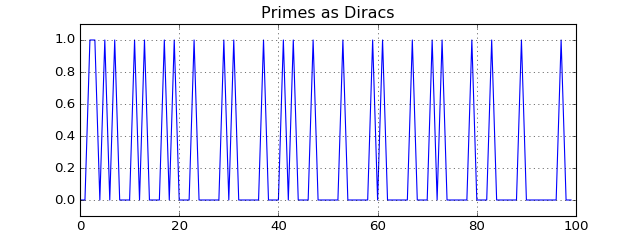# The Prime Numbers' Music, Staircase and Riemann Hypothesis¶

For DSP, mathematics is a fundamental tool to understand the different techniques. Hence, I'm also interested in different aspects of mathematics. And what aspect could be more "pure" mathematics than number theory and prime numbers? In this regard, to at least understand the still unsolved Riemann Hypothesis, where a price money of $1,000,000 will be given to the one who proves or disproves it, has been bothering me for quite some time. Despite a lot of material about the hypothesis being online, it was the book of Barry Mazur and William Stein entitled "Prime Numbers and the Riemann Hypothesis" which provided me at least a superficial understanding of it. The nice thing about this book is its very simple approach, requiring not more than high school math. Each page contains graphs and illustrations of the analyzed functions and hence makes it quite easy to follow. Accordingly, it's a very nice book to get to know a bit about the Riemann Hypothesis (RH). However, admittedly, the mathematical treatment is intentionally quite superficial. In this notebook, I do not want to directly talk about the RH. Instead, the notebook arose when I tried to replicate some calculations from the book. In particular, I was completely astonished, when I saw that the prime-counting function$\pi(x)$can be written as an infinite sum of continuous functions: $$\text{Number of primes below x}=\pi(x) = R_0(x)+\sum_{k=1}^{\infty} T_k(x)$$ where$R_0(x)$is some smooth function and the$T_k(x)$are oscillating correction terms which finally create the prime staircase. As the numerics seemed too straight-forward I sat down and just tried to replicate this calculation. However, it didn't work out directly and hence some more research was necessary. In this notebook, I want to describe my calculations. As a bonus, I give Marcus du Sautoy's title The Music of the Primes (which is another great to read book) a direct meaning, because we will actually hear how these correction functions sound. As a teaser, here are some sound samples. Scroll down to find out what they actually represent!   Tk(t), k=1  Tk(t), k=100  So, let's get started. For the calculations, I will make use of the mpmath library, which has a lot of number-theoretic functions already implemented. In particular, Riemann's$R(x)\$ function is already there. Please also dont care too much about mathematical exactness, we will just play around with the functions a bit.

import mpmath


Let's first calculate the first few prime numbers (to recap: a prime number is a number that is only divisible by 1 and itself):

N = 1000000
t = np.arange(0, N, 0.2)
primes = np.array(mpmath.mp.list_primes(N))
print ("First 20 prime numbers:")
print (primes[:20])

First 20 prime numbers:
[ 2  3  5  7 11 13 17 19 23 29 31 37 41 43 47 53 59 61 67 71]


Now, let's mark each prime number on the number line:

prime_diracs = np.zeros(N)
prime_diracs[primes] = 1
plt.plot(prime_diracs[:100])Nothing special here. Let's understand this number line as a time-domain function, which we can directly convert to sound. For comparison, we will also play white noise to see if the "music" is actually different from noise:

print ("White noise:")
display(Audio(data=np.random.randn(len(prime_diracs)), rate=44100))
print ("Primes as Diracs:")
display(Audio(data=prime_diracs, rate=44100))

White noise: